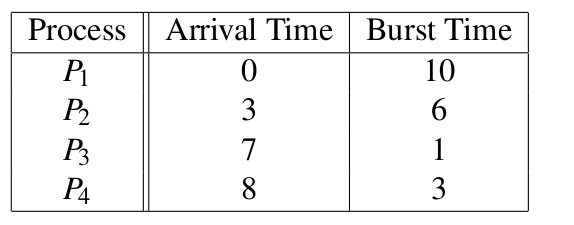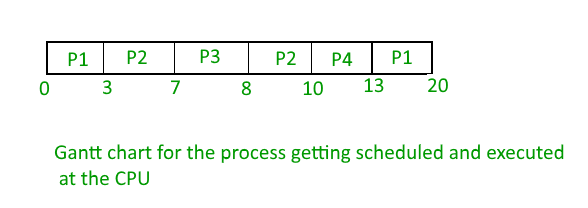# GATE | GATE-CS-2016 (Set 2) | Question 57

Consider the following processes, with the arrival time and the length of the CPU burst given in milliseconds. The scheduling algorithm used is preemptive shortest remaining-time first.The average turn around time of these processes is ___________ milliseconds.

(A) 8.25
(B) 10.25
(C) 6.35
(D) 4.25

Explanation: PreEmptive Shortest Remaining time first scheduling, i.e. that processes will be scheduled on the CPU which will be having least remaining burst time( required time at the CPU).

The processes are scheduled and executed as given in the below Gantt chart.Turn Around Time(TAT) = Completion Time(CT) – Arrival Time(AT)

TAT for P1 = 20 – 0 = 20

TAT for P2 = 10 – 3 = 7

TAT for P3 = 8- 7 = 1

TAT for P4 = 13 – 8 = 5

Hence, Average TAT = Total TAT of all the processes / no of processes = ( 20 + 7 + 1 + 5 ) / 4 = 33 / 4 = 8.25

Thus, A is the correct choice.

Quiz of this Question

My Personal Notes arrow_drop_up
Article Tags :

Be the First to upvote.

Please write to us at contribute@geeksforgeeks.org to report any issue with the above content.